## Originally Given November 5, 2002

1. Planes:

a) Find the equation of the plane that goes through the point (2,1,0) and has normal vector (2,1,-2).

b) Find the distance from the point (4,13,5) to the plane in part (a).

c) Find the equation of the plane that goes through the three points (2,1,0), (3,1,4) and (0,0,0) [No, this isn't the same plane as part a)].

d) Find the cosine of the angle between the planes of part (a) and (c).

2. Lines

a) Find the equation, in symmetric form, for the line through the point (2,1,0) in the direction (2,1,-2).

b) How far is the origin (0,0,0) from this line?

c) Find the equation, in symmetric form, for the line through the two points (2,1,0) and (3,-1,4).

d) Find the equation (in symmetric form) of the line through (2,1,0) that is perpendicular to the lines of parts (a) and (c).

3. Parametrized curves: Consider the parametrized curve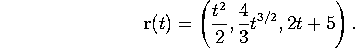a) Compute the position, velocity, unit tangent vector and speed at time t=1.

b) Compute the arc-length of the curve from t=0 to t=2.

4. Polar coordinates.

a) Sketch the curve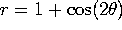. Mark clearly the angles (if any) where the curve goes through the origin, and the angles where r is maximal.

b) Find (in polar coordinates!) the points where this curve intersects the circle r=1/2.

c) Write down a definite integral that gives the area, in the first quadrant, inside the curve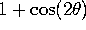but outside the circle r=1/2.

Extra credit) Evaluate this integral.

5. Partial derivatives. Consider the function of two variables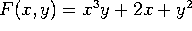.

a) Compute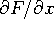and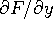and evaluate these at the point (1,1).

b) Use these to estimate the value of F(1.01, 1) and the value of F(1, 1.01).

c) Estimate the value of F(1.02, 1.01).

d) Compute the second-order partial derivatives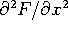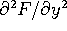, and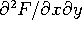.